# Calculating the probability for both teams to score in R

I recently read an interesting paper that was published last year, by Igor Barbosada Costa, Leandro Balby Marinho and Carlos Eduardo Santos Pires: Forecasting football results and exploiting betting markets: The case of “both teams to score. In the paper they try out different approaches for predicting the probability of both teams to score at least one goal (“both teams to score”, or BTTS for short). A really cool thing about the paper is that they actually used my goalmodel R package. This is the first time I have seen my package used in a paper, so im really excited bout that! In addition to using goalmodel, they also tried a few other machine learning approaches, where insted of trying to model the number of goals by using the Poisson distribution, they train the machine learning models directly on the both-teams-to-score outcome. They found that both approaches had similar performance.

I have to say that I personally prefer to model the scorelines directly using Poisson-like models, rather than trying to fit a classifier to the outcome you want to predict, whether it is over/under, win/draw/lose, or both-teams-to-score. Although I can’t say I have any particularly rational arguments in favour of the goal models, I like the fact that you can use the goal model to compute the probabilities of any outcome you want from the same model, without having to fit separate models for every outcome you are interested in. But then again, goalmodels are of course limited by the particlar model you choose (Poisson, Dixon-Coles etc), which could give less precice predictions on some of these secondary outcomes.

Okay, so how can you compute the probability of both teams to score using the Poisson based models from goalmodel? Here’s what the paper says: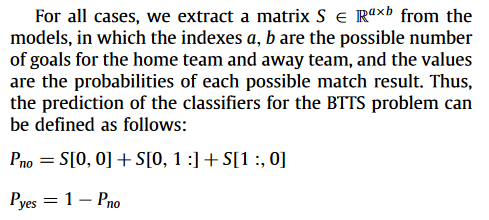This is a straightforward approach where they have the matrix with the probabilities of all possible scorelines, and then just add together the probabilities that correspond to the outcome of at least one team to score no goals. And since this is the exact opposite outcome (the complement) of both teams to score, you take one minus this probability to get the BTTS probability. I assume thay have used the predict_goals() function to get the matrix in question from a fitted goal model. In theory, the Poisson model allows to an infinite amount of goals, but in practice it is sufficient to just compute the matrix up to 10 or 15 goals.

Heres a small self-contained example of how the matrix with the score line probabilities is computed by the predict_goals() function, and how you can compute the BTTS probability from that.

# Expected goals by the the two oppsing teams.
expg1 <- 1.1
expg2 <- 1.9

# The upper limit of how many goals to compute probabilities for.
maxgoal <- 15

# The "S" matrix, which can also be computed by predict_goals().
# Assuming the independent Poisson model.
probmat <- dpois(0:maxgoal, expg1) %*% t(dpois(0:maxgoal, expg2))

# Compute the BTTS probability using the formula from the paper.
prob_btts <- 1 - (sum(probmat[2:nrow(probmat),1]) + sum(probmat[1,2:ncol(probmat)]) + probmat[1,1])


In this example the probability of both teams to score is 56.7%.

In many models, like the one used above, it is assumed that, the goal scoring probabilites for the two oppsing teams are statistically independent (given that you provide the expected goals). For this type of model there is a much simpler formula for computing the BTTS probability. Again, you compute the probability of at each of the teams to not score, and then take 1 minus these probabilities. And the probability of at least one team to score is just the product of the probability of both teams to score. In mathematical notation this is

$$P(BTTS) = (1 – P(X = 0)) \times (1 – P(Y = 0))$$

where X is the random variable for the number of goals scored by the first team, and Y is the corresponding random variable for the second team. The R code for this formula for the independent Poisson model is

prob_btts_2 <- (1 - dpois(0, lambda = expg1)) * (1 - dpois(0, lambda = expg2))


which you can verify gives the same result as the matrix-approach. This formula only works with the statistical independence assumption. The Dixon-Coles and bivariate Poisson (not in the goalmodel package) models are notable models that does not have this assumption, but instead have some dependence (or correlation) between the goal scoring probabilities for the two sides.

I have also found a relatively simple formula for BTTS probability for the Dixon-Coles model. Recall that the Dixon-Coles model applies an adjustment to low scoring outcomes (less than two goals scored by either team), shifting probabilities to (or from) 0-0 and 1-1 to 1-0 and 0-1 outcomes. The amount of probability that is shifted depends on the parameter called rho. For the BTTS probability, it is the adjustment to the probability of the 1-1 outcome that is of interest. The trick is basically to subtract the probability of the 1-1 outcome for the underlying independent model without the Dixon-Coles adjustment, and then add back the Dixon-Coles adjusted 1-1 probability. The Dixon-Coles adjustment for the 1-1 outcome is simply 1 – rho, which does not depend on the expected goals of the two sides.

Here is some R code that shows how to apply the adjustment:


rho <- 0.13

# 1-1 probability for the independent Poisson model.
p11 <- dpois(1, lambda = expg1) * dpois(1, lambda = expg2)

dc_correction <- (p11 * (1-rho)) - p11

# Apply the corrections
prob_btts_dc  <- prob_btts_2 + dc_correction


If you run this, you will see that the BTTS probability decreases to 55.4% when rho = 0.13.

I have added two functions for computing BTTS probabilities in the new version 0.6 of the goalmodel package, so be sure to check that out. The predict_btts() function works just like the other predict_* functions in the package, where you give the function a fitted goalmodel, together with the fixtures you want to predict, and it gives you the BTTS probability. The other function is pbtts(), which works independently of a fitted goalmodel. Instead you just give it the expected goals, and other paramters like the Dixon-Coles rho parameter, directly.

# Expected goals from over/under odds

I got a comment the other day asking about whether it is possible to get the expected number of goals scored from over/under odds, similar to how you can do this for odds for win, draw or lose outcomes. The over/under odds refer to the odds for the total score (the sum of the score for two opponents) being over or under a certain value, usually 2.5 in soccer.

It is possible, and rather easy even, to get the expected total score from the over/under odds, at least if you assume that the number of goals scored by the two teams follows a Poisson distribution. This is the same assumption that makes the method for extracting the expected goals from HDW odds possible. The Poisson distribution is really convenient and reasonable realistic probability model for different scorelines. It is controlled by a single parameter, called lambda, that is also the expected value (and the expected goals in this case). One convenient property of the Poisson is that the sum of two Poisson distributed variables with parameters lambda1 and lambda2 is also Poisson distributed, with the lambda being the sum of the two lambdas, i.e. lambdasum = lambda1 + lambda2.

So how can you find the expected total number of goals based on the over/under odds? First you need to convert the odds for the under outcome to a proper probability. How you do this depends on the format your odds come in, but in R you can use the odds.converter package to convert them to decimal format, and then use my own package called implied to convert them to proper probabilities.

After you have the probabilities for the under probability, you can use the Poisson formula to find the value of the parameter lambda that gives the probability for the under outcome that matched the probability from the odds. In R you can use the built-in ppois function to compute the probabilities for there being scored less than 2.5 goals when the expected total goals is 3.1 like this:

under <- 2.5
ppois(floor(under), lambda=3.1)


This will give us that the probability is 40.1% of two or less goals being scored in total, when the expected total is 3.1. Now you can try to manually adjust the lambda parameter until the output matches your probability from the odds. Another way is to automate this search using the built-in uniroot function. The uniroot function takes as input another function, and searches for the input value that gives the result 0. We therefore have to write a function that takes as input the expected goals, the probability implied by the odds, and the over/under limit, and returns the difference between the probability from the Poisson model and the odds probability. Here is one such function:

obj <- function(expg, under_prob, under){
(ppois(floor(under), lambda=expg) - under_prob)
}


Next we feed this to the uniroot function, and gives a realistic search interval for the expected goals, between 0.01 and 10 in this case, and the rest of the parameters. For this example I used 62% chance of there being scored less than 2.5 goals.

uniroot(f = obj,
interval = c(0.01, 10),
under_prob = 0.62,
under = 2.5)


From this I get that the expected total goals is 2.21.

You might wonder if it is possible to get the separate expected goals for the two teams from over/under odds using this method. This is unfortunately not possible. The only thing you can hope for is to get a range of possible values for the two expected goals that sums to the total expected goals. In our example with the expected total goals being 2.21, the range of possible values for the two expected goals can be plotted as a line like this: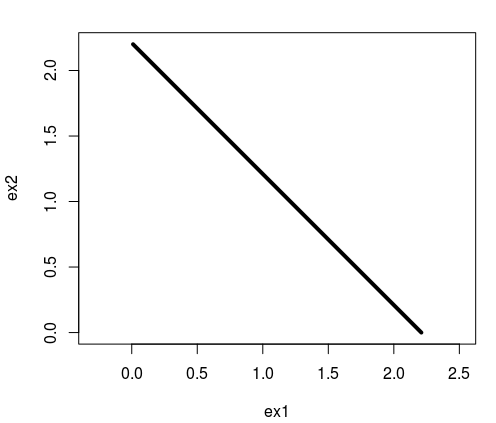If course, you can judge some pairs of expected goals being more likely than others, but there is no information about this in over/under odds alone. It might be possible, I am not 100% sure, that other non-Poisson models, which would involve more assumptions, could exploit the over/under odds to get expected goals for both teams.

# The probabilities implied by bookmaker odds: Introducing the ‘implied’ package

My package for converting bookmaker odds into probabilities is now on available from CRAN. The package contains several different conversion algorithms, which are all accessible via the implied_probabilities() function. I have written an introduction on how you can use the package here, together with a description of all the methods and with references to papers. But I also want to give some background to some of the methods here on the blog as well.

In statistics, an odd is usually taken to mean the inverse of a probability, that is 1/p, but in the betting world different odds formats exists. As usual, Wikipedia has a nice overview of the different formats. In the implied package, only inverse probability odds are allowed as inputs, which in betting are called decimal odds.

Now you might think that converting decimal odds to probabilities should be easy, you can just use the definition above and take the inverse of the odds to recover the probability. But it is not that simple, since in practice using this simple formula will give you improper probabilities. They will not sum to 1, as they should, but be slightly larger. This gives the bookmakers an edge and the probabilities (which aren’t real probabilities) can not be considered fair, and so different methods for correcting this exists.

Some methods uses different types of regression modelling combined with historical data to estimate the biases in the different outcomes. This is for example the case in the paper On determining probability forecasts from betting odds by Erik Štrumbelj. Anyway, the implied package does not include these kinds of methods. The reason I wanted to mention this paper is that this was where I first read about Shin’s method for the first time.

All the methods in the package are what I call one-shot methods. The conversion of a set of odds for a game only relies on the odds them self, and not on any other data. This is deliberate choice, since I didn’t want to make a modelling package, since that would be much more complicated.

Many of the methods in the package comes are described in the Wisdom of the Crowd document by Joseph Buchdahl, and a review paper by Clarke et al (Adjusting Bookmaker’s Odds to Allow for Overround).

Many of the methods in the package can be described as ad hoc methods. They basically use a simple mathematical formula that relates the true underlying probabilities to the improper probabilities given by the bookmakers odds. Then this formula is used to find the true probabilities so that they are proper (sum to 1) while also recovering the improper bookmaker probabilities.

A few other methods in the package are more theory based, like Shin’s method, and I find these methods really interesting. Shin’s method imagine that there are two types of bettors. The first type is the typical bettor, and the sum of bets by this type follows the “wisdom of the crowd” pattern which should reflect the true ncertainty of the outcome given the publicly available information. Then there is a second type of bettor, which has inside information and always bets on the winning outcome. However, the bookmaker don’t know what type of bettor the individual bettors are, and only observes the mixture of the two types. Here is the interesting part: By assuming the bookmakers know that there are two types of bettors, and that the bookmakers seek to maximize their profits, Shin was able to derive some complicated formulas that relate the true underlying “wisdom of the crowds” probabilities and the bookmakers odds. These formulas can be used in the same way as the ad hoc methods to find the underlying probabilities.

A natural question question is what method gives the most realistic probabilities? There is no definite answer to this, and different methods will be best in different markets and settings. You need to figure this out for yourself.

I am currently working on some new methods inspired by Shin’s framework which I hope to write about later. Shin’s work was mostly done in the context of horse racing, where there is realistic that some bettors have inside information. I hope to develop a method that is more relevant for football.

# Expected goals from bookmaker odds

I recently read an interesting paper called The Betting Odds Rating System: Using soccer forecasts to forecast soccer by Wunderlich and Memmert. In their paper they develop av variant of the good old Elo rating system. Instead of using the actual outcomes of each match to calculate the ratings, they use the probabilities of the outcomes, which they get from bookmaker odds.

I was wondering if a similar approach could be used together with the goalmodel package I released a couple of months ago. The models available in the package are models I have written about extensively on this blog, and they all work as follows: You use the number of goals scored to get some ratings of the goal scoring and goal conceding rates of each team. You then use these ratings to forecast the expected number of goals in the upcoming games. These expected goals can then be used to calculate the probabilities of the outcome (Home win, draw, away win). A crucial step in these calculations is the assumption that the number of goals scored follow the Poisson distribution (or some related distribution, like the Negative Binomial).

But can we turn this process the other way around, and use bookmaker odds (or odds from other sources) to get expected goals and maybe also attack and defense ratings like we do in the goalmodel package? I think this is possible. I have written a function in R that takes outcome probabilities and searches for a pair of expected goals that matches the probabilities. You can find it on github (Edit: The function is now included in the goalmodel package.). This function relies on using the Poisson distribution.

Next, I have expanded the functionality of the goalmodel package so that you can use expected goals for model fitting instead of just observed goals. This is possible by setting the model argument to “model = ‘gaussian'” or to “model = ‘ls'”. These two options are currently experimental, and are a bit unstable, so if you use them, make sure to check if the resulting parameter estimates make sense.

I used my implied package to convert bookmaker odds from the 2015-16 English Premier League into probabilities (using the power method), found the expected goals, and then fitted a goalmodel using the least squares method. Here are the resulting parameters, from both using the expected goals and observed goals:I wanted to use this season for comaprison as this was the season Leicester won unexpectedly, and in the Odds-Elo paper (figure 6) it seemed like the ratings based on the odds were more stable than the ones based on the actual results, which increased drastically during the season. In the attack and defense ratings from the goalmodels we see that Leicester have average ratings (which is what ratings close to 0 are) in the model based on odds, and much higher ratings based on the actual results. So the goalmodel and Elo ratings seem to agree, basically.

I also recently discovered another paper titled Combining historical data and bookmakers’odds in modelling football scores, that tries something similar as I have done here. They seem to do the same extraction of the expected goals from the bookmaker odds as I do, but they don’t provide the details. Instead of using the expected goals to fit a model, they fit a model based on actual scores (similar to what the goalmodel package do), and then they take a weighted average of the model based expected goals and the expected goals from the bookmaker odds.

# Introducing the goalmodel R package

I have written a lot about different models for forecasting football results, and provided a lot of R code along the way. Especially popular are my posts about the Dixon-Coles model, where people still post comments, four years since I first wrote them. Because of the interest in them, and the interest in some of the other models I have written about, I decided to tidy up my code and functions a bit, and make an R package out of it. The result is the goalmodel R package. The package let you fit the ordinary Poisson model, which was one of the first models I wrote about, the Dixon-Coles model, The Negative-Binomial model, and you can also use the adjustment I wrote about in my previous update.

The package contains a function to fit the different models, and you can even combine different aspects of the different models into the same model. You can for instance use the Dixon-Coles adjustment together with a negative binomial model. There is also a range of different methods for making prediction of different kinds, such as expected goals and over/under.

The package can be downloaded from github. It is still just the initial version, so there are probably some bugs and stuff to be sorted out, but go and try it out and let me know what you think!

# A simple re-implementation of the Dixon-Coles model

A couple of years ago I implemented the Dixon-Coles model for predicting football results here on this blog. That series of of blog posts is my most popular since I keep getting comments on it, some four years later.

One of the most common requests is advice on how to expand the model to include additional predictors. Unfortunately with the implementation I posted this was not so straightforward. It relied on some design matrices with dummy-coded variables, which is a standard way of doing things in regression modeling. The DC model isn’t a standard regression modeling problem, so using matrices complicated things. I posted some updates and variant across several posts, which in the end made the code a bit hard to follow and to modify.

Anyway, I’ve had a simpler implementation lying around for a while, and since there’s been far between updates on this blog lately I thought I’d post it.

First load some data from the engsoccerdata package. I’m going to use the 2011-12 season of the English Premier League, so the results can be compared with what I got from the first implementation.

library(dplyr)
library(engsoccerdata)

england %>%
filter(Season == 2011,
tier==1) %>%
mutate(home = as.character(home),
visitor = as.character(visitor))-> england_2011


Next we should create a list of initial parameter values. This will be used as a starting point for estimating the parameters. The list contains vectors of four groups of parameters, the attack and defense parameters of all teams, the home field advantage and the Dixon-Coles adjustment (rho). The attack and defense vector are named so that it is easy to look up the relevant parameter later on.

Notice also that a sum-to-zero constraint has to be added to the defense parameters, so in reality we are estimating one defense parameter less than the number of teams. Check this post for some more explanation of this.

# Make a vector of all team names.
all_teams <- sort(unique(c(england_2011$home, england_2011$visitor)), decreasing = FALSE)
n_teams <- length(all_teams)

# list of parameters with initial values.
parameter_list <- list(attack = rep(0.2, n_teams),
defense = rep(-0.01, n_teams-1),
home = 0.1,
rho= 0.00)

names(parameter_list$attack) <- all_teams names(parameter_list$defense) <- all_teams[-1] # the first parameter is computed from the rest.


Next we need a function that calculates the negative log-likelihood function, to be used with R’s built in optimizer.

One trick I use here is to relist the parameters. The optimizer want all parameter values as a single vector. When you have a lot of parameters that group together and is used in different parts of the model, this can quickly create some complicated indexing and stuff. By supplying the original parameter list, plus having named vectors, these problems essentially disappear.

Also notice how the expected goals are now simply computed by looking up the relevant parameters in the parameter list and adding them together. No need for matrix multiplications.

The Dixon-Coles adjustment function tau is the same as in the original implementation.

dc_negloglik <- function(params, goals_home, goals_visitor,
team_home, team_visitor, param_skeleton){

# relist, to make things easier.
plist <- relist(params, param_skeleton)

# There is a sum-to-zero constraint on defense parameters.
# The defense parameter for the first team is computed from the rest.
plist$defense <- c(sum(plist$defense)*-1, plist$defense) names(plist$defense) <- names(plist$attack) # add name to first element. # Home team expected goals lambda_home <- exp(plist$attack[team_home] + plist$defense[team_visitor] + plist$home)

# Away team expected goals
lambda_visitor <- exp(plist$attack[team_visitor] + plist$defense[team_home])

dc_adj <- tau(goals_home, goals_visitor, lambda_home, lambda_visitor, rho = plist$rho) # Trick to avoid warnings. if (any(dc_adj <= 0)){ return(Inf) } # The log-likelihood log_lik_home <- dpois(goals_home, lambda = lambda_home, log=TRUE) log_lik_visitor <- dpois(goals_visitor, lambda = lambda_visitor, log=TRUE) log_lik <- sum((log_lik_home + log_lik_visitor + log(dc_adj))) return(log_lik*-1) }  To actually estimate the parameters we feed the function, data and initial values to optim, and check the results.  optim_res <- optim(par = unlist(parameter_list), fn=dc_negloglik, goals_home = england_2011$hgoal,
goals_visitor = england_2011$vgoal, team_home = england_2011$home, team_visitor = england_2011$visitor, param_skeleton=parameter_list, method = 'BFGS') # relist, and calculate the remaining parameter. parameter_est <- relist(optim_res$par, parameter_list)
parameter_est$defense <- c( sum(parameter_est$defense) * -1, parameter_est$defense) names(parameter_est$defense) <- names(parameter_est\$attack)


I get the same home field advantage (0.27) and rho (-0.13) as in the original implementation. The other parameters differ, however. This is because of the sum-to-zero constraints are coded in a different way. This should not matter and both ways should give the same predictions.

I have not yet said anything about how to expand the model to include other predictors, but hopefully this implementation should make it easier. You can just add some new arguments to the dc_negloglik function that takes the variables in question as input, and add new parameter vectors to the parameter list as needed. Then the calculations of the expected goals should be modified to include the new parameters and predictors.

# Fitting Bradley-Terry models using Stan

I have recently played around with Stan, which is an excellent software to fit Bayesian models. It is similar to JAGS, which I have used before to fit some regression models for predicting football results. Stan differs from JAGS in a number of ways. Although there is some resemblance between the two, the model specification languages are not compatible with each other. Stan, for instance, uses static typing. On the algorithmic side, JAGS uses the Gibbs sampling technique to sample from the posterior; Stan does not do Gibbs sampling, but has two other sampling algorithms. In Stan you can also get point estimates by using built-in optimization routines that search for the maximum of the posterior distribution.

In this post I will implement the popular Bradley-Terry machine learning model in Stan and test it on some sports data (handball, to be specific).

The Bradley-Terry model is used for making predictions based on paired comparisons. A paired comparison in this context means that two things are compared, and one of them is deemed preferable or better than the other. This can for example occur when studying consumer preferences or ranking sport teams.

The Bradley-Terry model is really simple. Suppose two teams are playing against each other, then the probability that team i beats team j is

$$p(i > j) = \frac{r_i}{r_i + r_j}$$

where $$r_i$$ and $$r_j$$ are the ratings for the two teams, and should be positive numbers. It is these ratings we want to estimate.

A problem with the model above is that the ratings are not uniquely determined. To overcome this problem the parameters need to be constrained. The most common constraint is to add a sum-to-one constraint

$$\sum_k r_k = 1$$

I will explore a different constraint below.

Sine we are in a Bayesian setting we need to set a prior distribution for the rating parameters. Given the constraints that the parameters should be positive and sum-to-one the Dirichlet distribution is a natural choice of prior distribution.

$$r_1, r_2, …, r_p \sim Dir(\alpha_1, \alpha_2, …, \alpha_p)$$

where the hyperparameters $$\alpha$$ are positive real numbers. I will explore different choices of these below.

Here is the Stan code for the Bradley-Terry model:

data {
int<lower=0> N; // N games
int<lower=0> P; // P teams

// Each team is referred to by an integer that acts as an index for the ratings vector.
int team1[N]; // Indicator arrays for team 1
int team2[N]; // Indicator arrays for team 1
int results[N]; // Results. 1 if team 1 won, 0 if team 2 won.

vector[P] alpha; // Parameters for Dirichlet prior.
}

parameters {
// Vector of ratings for each team.
// The simplex constrains the ratings to sum to 1
simplex[P] ratings;
}

model {
real p1_win[N]; // Win probabilities for player 1

ratings ~ dirichlet(alpha); // Dirichlet prior.

for (i in 1:N){
p1_win[i] = ratings[team1[i]] / (ratings[team1[i]] + ratings[team2[i]]);
results[i] ~ bernoulli(p1_win[i]);
}
}


The way I implemented the model you need to supply the hyperparameters for the Dirichlet prior via R (or whatever interface you use to run Stan). The match outcomes should be coded as 1 if team 1 won, 0 if team 2 won. The two variables team1 and team2 are vectors of integers that are used to reference the corresponding parameters in the ratings parameter vector.

Before we fit the model to some data we need to consider what values we should give to the hyperparameters. Each of the parameters of the Dirichlet distribution corresponds to the rating of a specific team. Both the absolute magnitude and the relative magnitudes are important to consider. A simple case is when all hyperparameters have the same value. Setting all hyperparameters to be equal to each other, with a value greater or equal to 1, implies a prior belief that all the ratings are the same. If they are between 0 and 1, the prior belief is that the ratings are really really different. The magnitude also plays a role here. The greater the magnitudes are, the stronger the prior belief that the ratings are the same.

Let’s fit some data to the model. Below are the results from fitting the results from 104 games from the 2016-17 season of the Norwegian women’s handball league, with 11 participating teams. I had to exclude six games that ended in a tie, since that kind of result is not supported by the Bradley-Terry model. Extension exists that handle this, but that will be for another time.

Below are the results of fitting the model with different sets of priors, together with the league points for comparison. For this purpose I didn’t do any MCMC sampling, I only found the MAP estimates using the optimization procedures in Stan.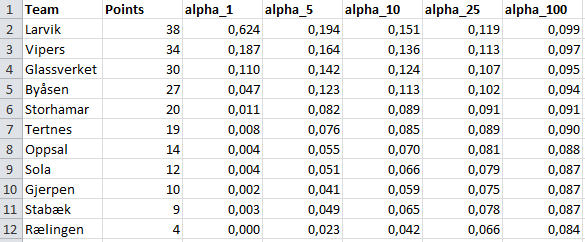For all the priors the resulting ratings give the same ranking. This ranking also corresponds well with the ranking given by the league points, except for Gjerpen and Stabæk which have switched place. We also clearly see the effect of the magnitude of the hyperparameters. When all the $$\alpha$$‘s are 1 the ratings varies from almost 0 to about 0.6. When they are all set to 100 the ratings are almost all the same. If these ratings were used to predict the result of future matches the magnitudes of the hyperparameters could be tuned using cross validation to find the value that give best predictions.

What if we used a different hyperparameter for each team? Below are the results when I set all $$\alpha$$‘s to 10, except for the one corresponding to the rating for Glassverket, which I set to 25. We clearly see the impact. Glassverket is now considered to be the best team. This is nice since it demonstrates how actual prior information, if available, can be used to estimate the ratings.I also want to mention another way to fit the Bradley-Terry model, but without the sum-to-one constraint. The way to do this is by using a technique that the Stan manual calls soft centering. Instead of having a Dirichlet prior which enforces the constraint, we use a normal distribution prior. This prior will not give strict bounds on the parameter values, but will essentially provide a range of probable values they can take. For the model I chose a prior with mean 20 and standard deviation 6.

$$r_1, r_2, …, r_p \sim N(\mu = 20, \sigma = 6)$$

The mean hyperprior here is arbitrary, but the standard deviation required some more considerations. I reasoned that the best team would probably be in the top 99 percentile of the distribution, approximately three standard deviations above the mean. In this case this would imply a rating of 20 + 3*6 = 38. Similarly, the worst team would probably be rated three standard deviations below the mean, giving a rating of 2. This implies that the best team has a 95% chance of winning against the worst team.

Here is the Stan code:

data {
int<lower=0> N;
int<lower=0> P;

int team1[N];
int team2[N];
int results[N];

real<lower=0> prior_mean; // sets the (arbitrary) location of the ratings.
real<lower=0> prior_sd; // sets the (arbitrary) scale of the ratings.

}

parameters {
real<lower=0> ratings[P];
}

model {
real p1_win[N];

// soft centering (see stan manual 8.7)
ratings ~ normal(prior_mean, prior_sd);

for (i in 1:N){
p1_win[i] = ratings[team1[i]] / (ratings[team1[i]] + ratings[team2[i]]);
results[i] ~ bernoulli(p1_win[i]);
}
}


And here are the ratings for the handball teams. The ratings are now on a different scale than before and largely matches the prior distribution. The ranking given by this model agrees with the model with the Dirichlet prior, with Gjerpen and Stabek switched relative to the league ranking.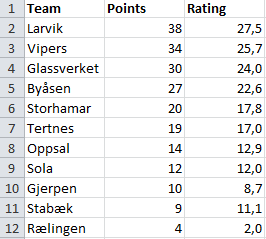# Which model is the best?

I had a discussion on Twitter a couple of weeks ago about which model is the best for predicting football results. I have suspected that the Dixon & Coles model (DC), which is a modification of the Poisson model, tend to overfit. Hence it should not generalize well and give poorer predictions. I have written about one other alternative to the Poisson model, namely the Conway-Maxwell Poisson model (COMP). This is a model for count data that can be both over-, equi- and underdispersed. It is basically a Poisson model but without the assumption that the variance equals the mean. I have previously done some simple analyses comparing the Poisson, DC and COMP models, and concluded then that the COMP model was superior. The analysis was however a bit to simple, so I have now done a more proper evaluation of the models.

A proper way to evaluatie the models is to do a backtest. For each day there is a game played, the three models are fitted to the available historical data (but not data from the future, that would be cheating) and then used to predict the match outcomes for that day. I did this for two leagues, the English Premier League and German Bundesliga. The models were fitted to data from both the top league and the second tier divisions, since this improves the models, but only the results of the top division was predicted and used in the evaluation. I used a separate home field advantage for the two divisions and the rho parameter in the DC model and the dispersion parameter in the COMP model was estimated using the top division only.

To measure the model’s predictive ability I used the Ranked Probability Score (RPS). This is the proper measure to evaluate predictions for the match outcome in the form of probabilities for home win, draw and away win. The range of the RPS goes from 0 (best possible predictions) to 1 (worst possible prediction). Since the three models actually model the number of goals, I also looked at the probability they gave for the actual score.

For all three models I used the Dixon & Coles method to weight the historical data that is used in training the models. This requires tuning. For both the English and German leagues I backtested the models on different values of the weighting parameter $$\xi$$ on the seasons from 2005-06 to 2009-10, with historical data available from 1995. I then used the optimal $$\xi$$ for backtesting the seasons 2010-11 up to December 2016. This last validation period covers 1980 Bundesliga matches and 2426 Premier League matches.

Here are the RPS for the three models plottet against $$\xi$$. Lower RPS is better and lower $$\xi$$ weights more recent data higher.The graphs show a couple of things. First, all three models have best predictive ability at the same value of $$\xi$$, and that they compare similarly also for non-optimal values of $$\xi$$. This makes things a bit easier since we don’t have to worry that a different value of $$\xi$$ will alter our evaluations about which model is the best.

Second, there is quite some difference between the models for the German and English data. In the English data the COMP model is clearly best, while the DC is the worst. In the German league, the DC is clearly better, and the COMP and Poisson models are pretty much equally good.

So I used the optimal values of $$\xi$$ (0.0021 and 0.0015 for Premier League and Bundesliga, respectively) to validate the models in the data from 2010 and onwards.

Here is a table of the mean RPS for the three models: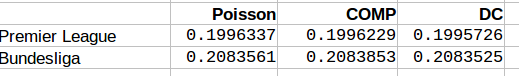We see that for the both English Premier League and German Bundesliga the DC model offers best predictions. The COMP model comes second in Premier League, but has worst performance in the Bundesliga. It is interesting that the DC model performed worst in the tuning period for the Premier League, now was the best one. For the Bundesliga the models compared similarly as in the tuning period.

I also looked at how often the DC and COMP models had lower RPS than the Poisson model. The results are in this table:The COMP model outperformed the Poisson model in more than 60% of the matches in both leagues, while the DC model did so only about 40% of the time.

When looking at the goal scoring probabilities. Here is a table of the sum of the minus log probabilities for the actual scoreline. Here a lower number also indicates better predictions.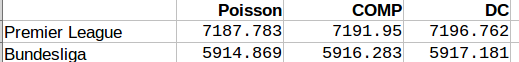Inn both the Premier League and Bundesliga the Poisson model was best, followed by COMP, with the DC model last.

We can also take a look at the parameter values for the extra parameters the DC and COMP models has. Remember that the DC models is becomes the Poisson model when rho = 0, while the COMP model is the same as the Poisson model when upsilon = 1, and is underdispersed when upsilon is greater than 1.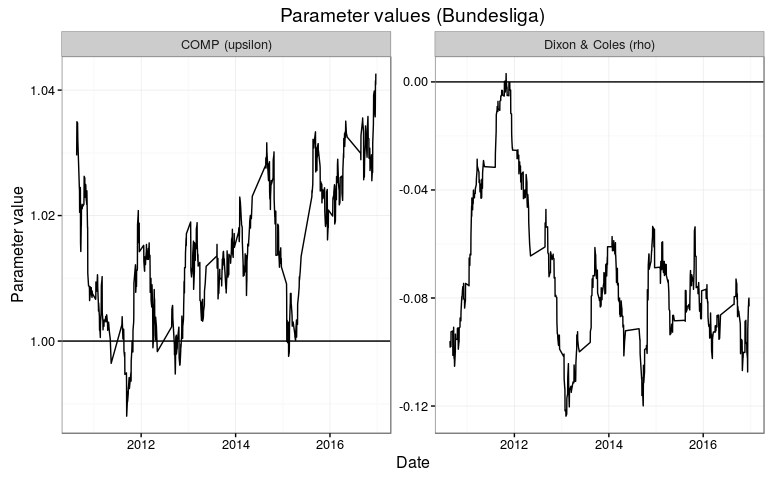The parameter estimates fluctuates a bit. It is intersting to see that the rho parameter in the DC model tend to be below 1, which gives the opposite direction of what Dixon and Coles found in their 1997 paper. In the Premier League, the parmater makes a big jump to above 0 at the end of the 2013-14 season. The parameter appears to be a bit more consistent in the Bundesliga, but also there we see a short period where the parameter is around 0.

The dispseriosn parameter upsilon also isn’t all that consistent. It is generally closer to 1 in the Bundesliga than in the Premier League. I think this is consistent with why this model was better in the Premier League than in the Bundesliga.

All inn all I think it is hard to conclude which of the three models is the best. The COMP and DC models both adjusts the Poisson model in their own specific ways, and this may explain why the different ways of measuring their predictive abilities are so inconsistent. The DC model seem to be better in the German Bundesliga than in the English Premier League. I don’t think any of the two models are generally better than the ordinary Poisson model, but it could be worthwhile to look more into when the two models are better, and perhaps they could be combined?

# Calculate the ranked probability score in R

I was asked in the comments for the R code for the ranked probability score, so instead of posting it deep down in the comments I thought I’d post it as a proper blog instead. The ranked probability score (RPS) is a measure of how similar two probability distributions are and is used as a way to evaluate the quality of a probabilistic prediction. It is an example of a proper scoring rule.

The RPS was brought to my attention in the paper Solving the problem of inadequate scoring rules for assessing probabilistic football forecasting models by Constantinou and Fenton. In that paper they argue that the RPS is the best measure of the quality of football predictions when the predictions are of the type where you have probabilities for the outcome (home win, draw or away win). The thing about the RPS is that it also reflects that an away win is in a sense closer to a draw than a home win. That means that a higher probability predicted for a draw is considered better than a higher probability for home win if the actual result is an away win.

You can also find some more details at the pena.lt/y blog.

The following R function takes two arguments. The first argument (predictions) is a matrix with the predictions. It should be laid out so that each row is one prediction, laid out in the proper order, where each element is a probability and each row sum to 1. The second argument (observed) is a numeric vector that indicates which outcome that was actually observed.

For assessing football predictions the predictions matrix would have three columns, with the probabilities for the match ordered as home, draw and away (or in the opposite order).

rankProbScore <- function(predictions, observed){
ncat <- ncol(predictions)
npred <- nrow(predictions)

rps <- numeric(npred)

for (rr in 1:npred){
obsvec <- rep(0, ncat)
obsvec[observed[rr]] <- 1
cumulative <- 0
for (i in 1:ncat){
cumulative <- cumulative + (sum(predictions[rr,1:i]) - sum(obsvec[1:i]))^2
}
rps[rr] <- (1/(ncat-1))*cumulative
}
return(rps)
}


# Underdispersed Poisson alternatives seem to be better at predicting football results

In the previous post I discussed some Poisson-like probability distributions that offer more flexibility than the Poisson distribution. They typically have an extra parameter that controls the variance, or dispersion. The reason I looked into these distributions was of course to see if they could be useful for modeling and predicting football results. I hoped in particular that the distributions that can be underdispersed would be most useful. If the underdispersed distributions describe the data well then the model should predict the outcome of a match better than the ordinary Poisson model.

The model I use is basically the same as the independent Poisson regression model, except that the part with the Poisson distribution is replaced by one of the alternative distributions. Let the $$Y_{ij}$$ be the number of goals scored in game i by team j

$$Y_{ij} \sim f(\mu_{ij}, \sigma)$$
$$log(\mu_{ij}) = \gamma + \alpha_j + \beta_k$$

where $$\alpha_j$$ is the attack parameter for team j, and $$\beta_k$$ is the defense parameter for opposing team k, and $$\gamma$$ is the home field advantage parameter that is applied only if team j plays at home. $$f(\mu_{ij}, \sigma)$$ is one of the probability distributions discussed in the last post, parameterized by the location parameter mu and dispersion parameter sigma.

To these models I fitted data from English Premier League from the 2010-11 season to the 2014-15 season. I also used Bundesliga data from the same seasons. The models were fitted separately for each season and compared to each other with AIC. I consider this only a preliminary analysis and I have therefore not done a full scale testing of the accuracy of predictions where I refit the model before each match day and use Dixon-Coles weighting.

The five probability distributions I used in the above model was the Poisson (PO), negative binomial (NBI), double Poisson (DPO), Conway-Maxwell Poisson (COM) and the Delaporte (DEL) which I did not mention in the last post. All of these, except the Conway-Maxwell Poisson, were easy to fit using the gamlss R package. I also tried two other gamlss-supported models, the Poisson inverse Gaussian and Waring distributions, but the fitting algorithm did not work properly. To fit the Conway-Maxwell Poisson model I used the CompGLM package. For good measure I also fitted the data to the Dixon-Coles bivariate Poisson model (DC). This model is a bit different from the rest of the models, but since I have written about it before and never really tested it I thought this was a nice opportunity to do just that.

The AIC calculated from each model fitted to the data is listed in the following table. A lower AIC indicates that the model is better. I have indicated the best model for each data set in red.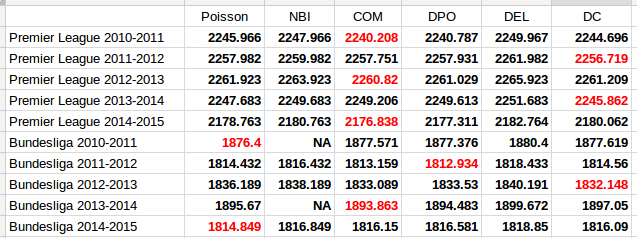The first thing to notice is that the two models that only account for overdispersion, the Negative Binomial and Delaporte, are never better than the ordinary Poisson model. The other and more interesting thing to note, is that the Conway-Maxwell and Double Poisson models are almost always better than the ordinary Poisson model. The Dixon-Coles model is also the best model for three of the data sets.

It is of course necessary to take a look at the estimates of the parameters that extends the three models from the Poisson model, the $$\sigma$$ parameter for the Conway-Maxwell and double Poisson and the $$\rho$$ for the Dixon-Coles model. Remember that for the Conway-Maxwell a $$\sigma$$ greater than 1 indicates underdispersion, while for the Double Poisson model a $$\sigma$$ less than 1 is indicates underdispersion. For the Dixon-Coles model a $$\rho$$ less than 0 indicates an excess of 0-0 and 1-1 scores and fewer 0-1 and 1-0 scores, while it is the opposite for $$\rho$$ greater than 0.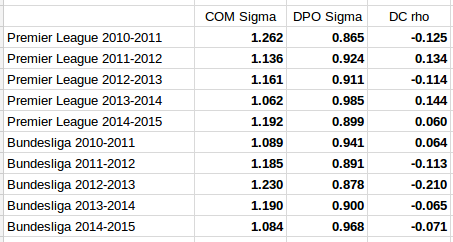It is interesting to see that the estimated dispersion parameters indicate underdispersion for all the data sets. It is also interesting to see that the data sets where the parameter estimates are most indicative of equidispersion is where the Poisson model is best according to AIC (Premier League 2013-14 and Bundesliga 2010-11 and 2014-15).

The parameter estimates for the Dixon-Coles model do not give a very consistent picture. The sign seem to change a lot from season to season for the Premier League data, and for the data sets where the Dixon-Coles model was found to be best, the signs were in the opposite direction of what where the motivation described in the original 1997 paper. Although it does not look so bad for the Bundesliga data, this makes me suspect that the Dixon-Coles model is prone to overfitting. Compared to the Conway-Maxwell and double Poisson models that can capture more general patterns in all of the data, the Dixon-Coles model extends the Poisson model to just parts of the data, the low scoring outcomes.

It would be interesting to do fuller tests of the prediction accuracy of these three models compared to the ordinary Poisson model.